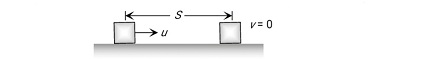# And due to friction, it comes to rest after covering a distance S. Then find S.

For the below figureS is given by

where

coefficient of friction

S = distance traveled

g = gravity

u=initial velocity

Exams
Articles
Questions